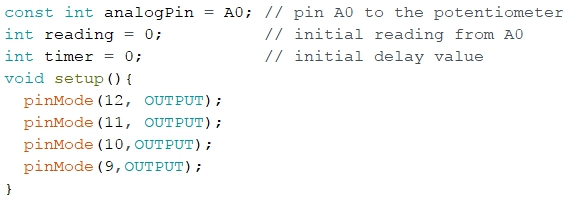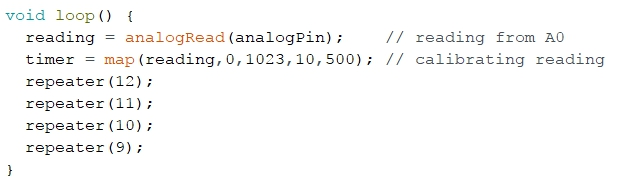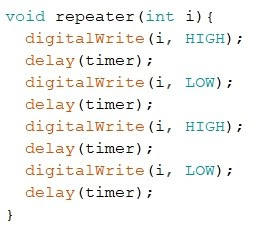## Tuesday, July 11, 2017

### 7 - Arduino: strobe light effect

ARDUINO: STROBE LIGHT EFFECT

- Layout for this exercise:1 - Introduction

- The objective of this exercise is to achieve the light effect of strobe based on emitting flashing light by 8 red and yellow LEDs, simulating the flag of Spain.

- The strobe light effect is a visual phenomenon caused by aliasing that occurs when continuous motion is represented by a series of short or instantaneous samples.

- It occurs when the view of a moving object or blinking light is represented by a series of short samples as distinct from a continuous view at a rate close to the sampling rate.

https://en.wikipedia.org/wiki/Stroboscopic_effect

- The schematics of this exercise is an Arduino board connected to 8 LEDs and a potentiometer connected to the analog pin A0.

2 - Code

- The code for this exercise:- Let's analyze the code.

- Setting the pins for the couple of LEDs (digital pins 12,11,10 and 9) and the potentiometer (analog pin A0):- The readings on the A0 are calibrated from 0 to 1023 giving an interval of 10 to 500 ms, what is a time enough short so that the human eye perceives the light as an overlay of flashes leading to the strobe effect.- As seen in the previous section the function repeater is applied to the digital pins 12, 11, 10 and  9:3 - Testing the circuit

- The video shows how the blinking light speed is controlled by the potentiometer in the interval of 10 to 500 ms, simulating the flashing colors of the Spanish flag: